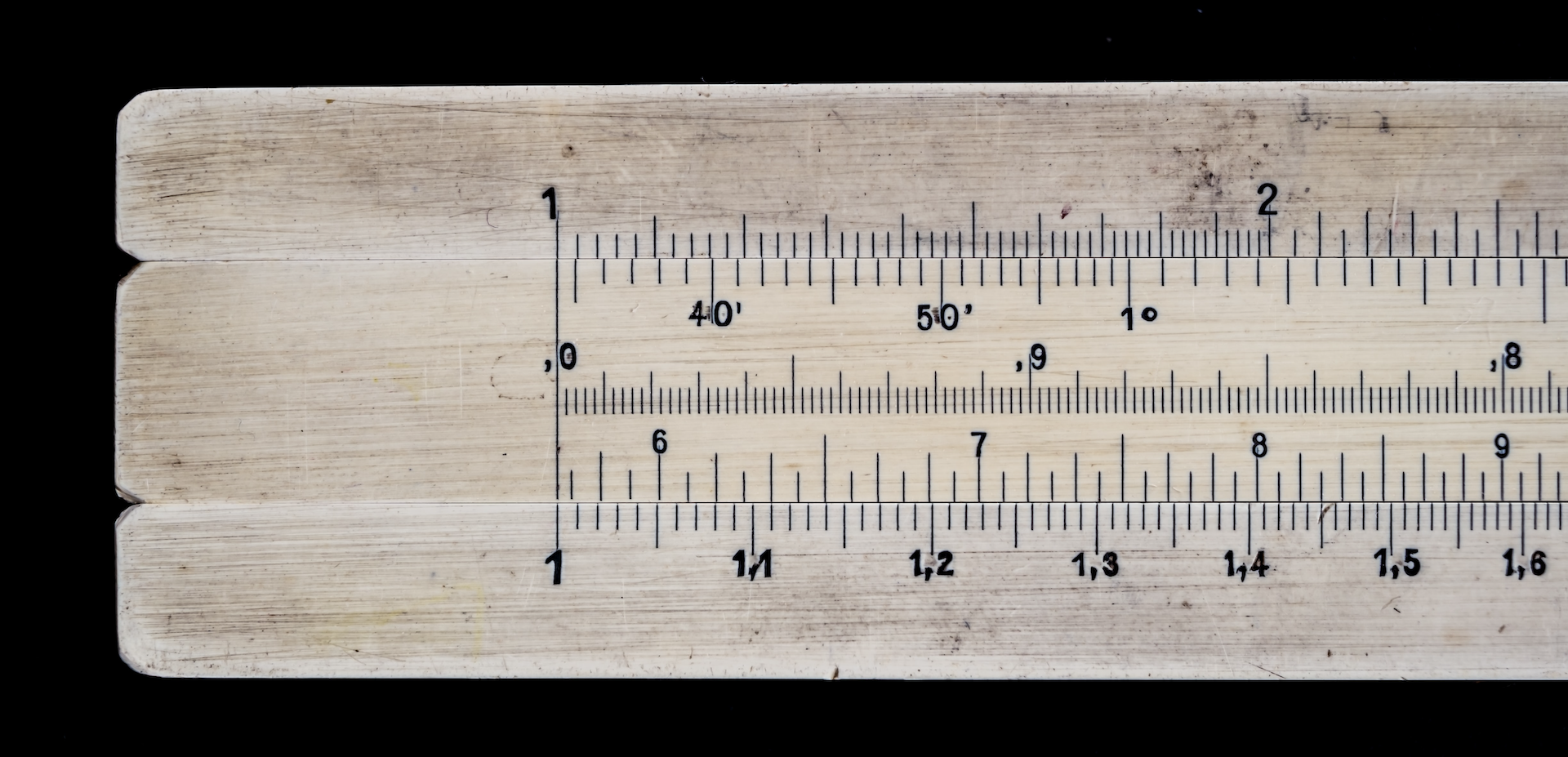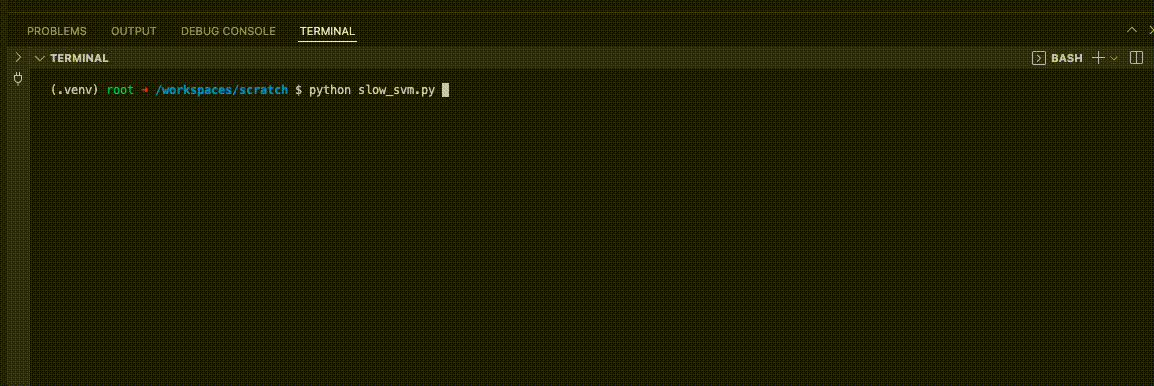Photo by Roberto Sorin on Unsplash

# The log10 of 0 is over 9000… right?

##### Applying log10 with numpy on a subset of values, and why you should always add ‘out’ to numpy functions when using a where ufuncThom Hopmans
Mar 30, 2022
datascience python practices numpy

Recently, one of our models was taking a very long time to fit after adding a new feature. This was unexpected, as on previous occasions the model fitting process was actually quite fast. The model in question was a linear SVM and we could see it was not converging anymore when fitting the model. The first thing to check for with linear SVMs when this happens is numerical or scaling issues in the training dataset, as libSVM is in theory guaranteed to converge (source). Adding the new feature indeed resulted in numerical issues, i.e. a few values greater than 100.000 were remaining in the dataset after scaling. These values were dominating all other, much smaller, numerical values.Figure 1 – Example of a linear SVM not converging

## What went wrong?

So why did the feature not scale properly? After all, our applied (and preferred) preprocessing method was to apply a clip negative (i.e. clip `x` to `[0, inf)`) followed by a log10 transformation to scale the model feature to a small interval. Needless to say, a tedious debugging process followed, where at some point even the term Heisenbug was used. Eventually, the culprit was found: the problem was the line where we apply numpy’s log10 function.

The logarithm function is undefined for 0. So log10 was only applied on strictly positive values using `np.log10(arr, where=(arr > 0))`. Leaving all zero values untouched we expected this to be a well-defined transformation, i.e. no runtime warnings from applying log10 on zero.

Unfortunately, this implementation of `where` in numpy statements can lead to unexpected behaviour, because we did not add an `out` argument. I hear you thinking… why do we need `out`? Well, the numpy docs state the following:

where: This condition is broadcast over the input. At locations where the condition is True, the out array will be set to the ufunc result. Elsewhere, the out array will retain its original value. Note that if an uninitialized out array is created via the default out=None, locations within it where the condition is False will remain uninitialized.

Those uninitialized values can then be whatever value was in there previously! For example, a value from a completely different model feature on which the log10 was also applied. Makes sense, right? If you find this hard to believe, you can try it yourself using the following code snippet or on Deepnote. More about arrays without initializing entries, including examples, can be found in the numpy empty() docs.

``````import numpy as np
print(f"Numpy version: {np.__version__}")

abc = np.array([0.00000, 100, 1000])
print(f"Initialize numpy array: {abc}")

# Random transformation to make the next transformation go rogue
np.log10(abc)

# Unexpected result
print("Expected result after transformation: [0. 2. 3.]")
print("Actual result after transformation:", np.log10(abc, where=(abc>0.0)))

>>> Numpy version: 1.21.5
>>> Initialize numpy array: [ 0. 100. 1000.]
>>> Expected result after transformation: [0. 2. 3.]
>>> Actual result after transformation: [-inf 2. 3.]
``````

## How to deal with the problem?

The solution in our case is simple though: we follow the docs. We initialize a new array full of zeros and set it in `out`. That way, all values that are untouched by our where clause, i.e. those greater than zero, are still initialized as zeros. If we want to use `where > 10` our initialization would be a bit more complex, but still manageable. The code snippet below (or on Deepnote) shows we now get the expected result, hurray! 🎉

``````import numpy as np
print(f"Numpy version: {np.__version__}")

abc = np.array([0.00000, 100, 1000])
print(f"Initialize numpy array: {abc}")

# Random transformation to make the next transformation go rogue
np.log10(abc)

# Good result
print("Expected result after transformation: [0. 2. 3.]")
print(
"Actual result after transformation:",
np.log10(abc, out=np.zeros(abc.shape), where=(abc > 0.0)),
)

>>> Numpy version: 1.21.5
>>> Initialize numpy array: [   0.  100. 1000.]
>>> Expected result after transformation: [0. 2. 3.]
>>> Actual result after transformation: [0. 2. 3.]
``````

Therefore, the proper way to use `where` (or other ufuncs) in numpy is by explicitly initializing an output array, i.e.

Now you also know!

## More from Floryn

Floryn is a fast growing Dutch fintech, we provide loans to companies with the best customer experience and service, completely online. We use our own bespoke credit models built on banking data, supported by AI & Machine Learning.

###### Topics
© 2021 Floryn B.V.